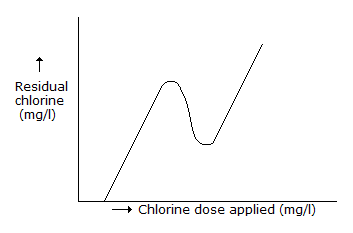# Civil Engineering - UPSC Civil Service Exam Questions

31.

Consider the following oxides :
1. Al2O3
2. CaO
3. SiO2
The correct sequence in increasing order of their percentage in an ordinary portland cement is

 A. 2, 1, 3 B. 1, 3, 2 C. 3, 1, 2 D. 1, 2, 3

Explanation:

No answer description available for this question. Let us discuss.

32.

The modules of elasticity (E) of concrete is given by

 A. E = 1000 fck B. E = 5700 fck C. E = 5700 fck D. E = 10, 000 fck

Explanation:

No answer description available for this question. Let us discuss.

33.

A 6 hour storm has 6 cm of rainfall and the resulting runoff was 3 cm. If φ = index remains at the same value, which one of the following is the runoff due to 12 cm of rainfall in 9 hours in the catchment?

 A. 4.5 cm B. 6.0 cm C. 7.5 cm D. 9.0 cm

Explanation:

No answer description available for this question. Let us discuss.

34.

In the plot of residual chlorine versus chlorine dose applied shown in the above figure, the curve will not have any (0, 0) point becauseA. of experimental error B. chlorine escapes into the atmosphere C. chlorine requires some contact time D. chlorine is consumed for disinfection

Explanation:

No answer description available for this question. Let us discuss.

35.

Which of the following pairs regarding the defects in timber are correctly matched ?
1. Upsets....due to overmaturity and unventilated star age of wood.
2. Fwciness....due to crushing of fibres running transversel.
3. Star shakes....radial splits widest at the circumference and diminishing towards the centre.
4. Heart shakes....cracks widest at the centre and diminishing towards the outer circumference.
Select the correct answer using the codes given below.

 A. 1 and 2 B. 3 and 4 C. 1 and 4 D. 2 and 4